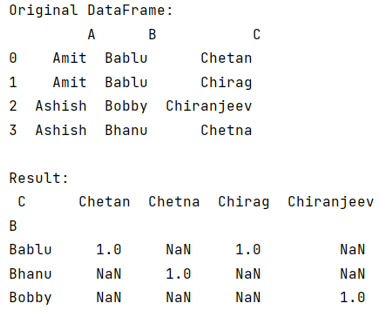# Python Pandas: Pivot table with aggfunc = count unique distinct

Given a DataFrame, we need to pivot table with aggfunc=count unique distinct. Submitted by Pranit Sharma, on July 23, 2022

Pandas is a special tool that allows us to perform complex manipulations of data effectively and efficiently. Inside pandas, we mostly deal with a dataset in the form of DataFrame. DataFrames are 2-dimensional data structures in pandas. DataFrames consist of rows, columns, and data.

Many times, for a better understanding of datasets or to analyze the data according to our compatibility, we need to reorder or reshape the given DataFrame according to index and column values. pandas.DataFrame.pivot() helps us to achieve this task.

## Pandas pivot table count frequency in one column

To use a pivot table with aggfunc (count), for this purpose, we will use pandas.DataFrame.pivot() in which we will pass values, index, and columns as a parameter also we will pass a parameter called aggfunc.

This method is used to reshape the given DataFrame according to index and column values. It is used when we have multiple items in a column, we can reshape the DataFrame in such a way that all the multiple values fall under one single index or row, similarly, we can convert these multiple values as columns.

Let us understand with the help of an example,

## Python program for pivot table with aggfunc=count unique distinct

```# Importing pandas package
import pandas as pd

# Creating a Dictionary
d={
'A' : ['Amit', 'Amit', 'Ashish', 'Ashish'],
'B' : ['Bablu', 'Bablu', 'Bobby', 'Bhanu'],
'C' : ['Chetan', 'Chirag', 'Chiranjeev', 'Chetna']
}

# Creating a DataFrame
df = pd.DataFrame(d)

# Display original DataFrame
print("Original DataFrame:\n",df,"\n")

# Using pivot table
result = df.pivot_table(values='A', index='B', columns='C', aggfunc=lambda x: len(x.unique()))

# Display result
print("Result:\n",result)
```

## Output